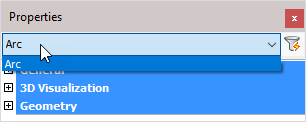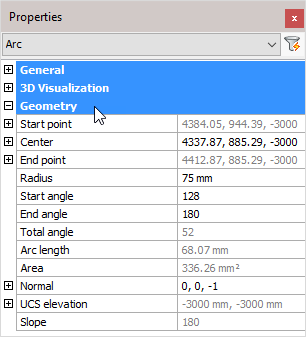The 'Arc' object type has the following headings:

General

3D Visualization

GeometryGeneral: Is common to all object types, and covers general AutoCAD and BricsCAD settings, including: Color / Layer / Linetype / Linetype scale / Plot style / Lineweight / Transparency / Hyperlink / Handle /

3D Visualization: Is also common to all object types and includes: Material.

The material that is referred to here is only the visual representation material. For changing the actual material of parts, see the Additional Data section of plates, profiles or structures.

Geometry:Note! - * indicates that the given value is a resultant of the sum of other values in the panel - and cannot be individually edited.

Start point: * Start point of the arc – expressed as the X, Y, and Z coordinates

Center: The geometric center of the arc – expressed as the X, Y, and Z coordinates

End point: * End point of the arc – expressed as the X, Y, and Z coordinates

Start angle: Measured from the horizontal plane

End angle: Measured from the horizontal plane

Total angle: * = (End angle - start angle)

Arc Length: * Calculated arc length

Area: * calculated area

Normal: Determines the 3D unit normal vector of a selected circle, arc, or PolyLine arc segment. This normal vector is the Z coordinate of the object coordinate system (OCS) of the selected object.

UCS elevation: * UCS elevation (Reference)

Slope: * Slope angle relative to the horizontal plane (Reference)